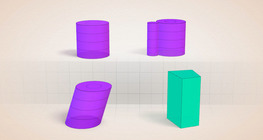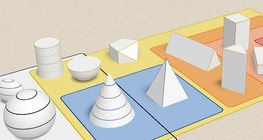Дана: 0

Барлыгы: 0,00

# Császár polyhedron

### Császár polyhedron

The Császár polyhedron is a nonconvex polyhedron with 14 triangular faces.

Математика

Этикеткалар

Император полиэдра, face, vertex, polyhedrons, neighbouring vertex, solids, diagonal, математика

### Байланысты экстралар#### Szilassi polyhedron

This special concave polyhedron was named after a Hungarian mathematician.#### Conic solids

This animation demonstrates various types of cones and pyramids.#### Cylindrical solids

This animation demonstrates various types of cylindrical solids as well as their lateral...#### Euler's polyhedron formula

The theorem formulated by Leonhard Euler describes one of the basic properties of convex...#### Sphere

A sphere is the set of points which are all within the same distance from a given point...#### Non-orientable surfaces

The Möbius strip and the Klein bottle are special two-dimensional surfaces with only one...#### Grouping of solids 1

This animation demonstrates various groups of solids through examples.#### Grouping of solids 2

This animation demonstrates various groups of solids through examples.#### Grouping of solids 3

This animation demonstrates various groups of solids through examples.#### Grouping of solids 4

This animation demonstrates various groups of solids through examples.#### Platonic solids

This animation demonstrates the five regular three-dimensional (or Platonic) solids, the...#### Shape games

Find views and nets of three-dimensional shapes.